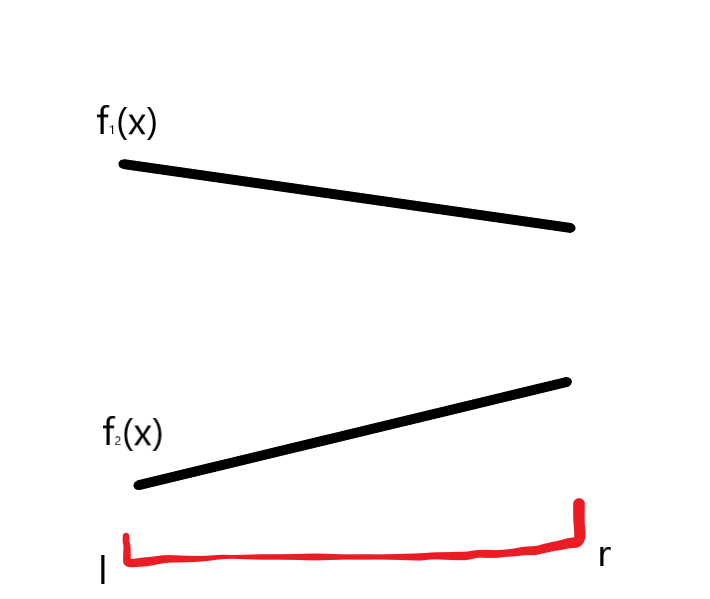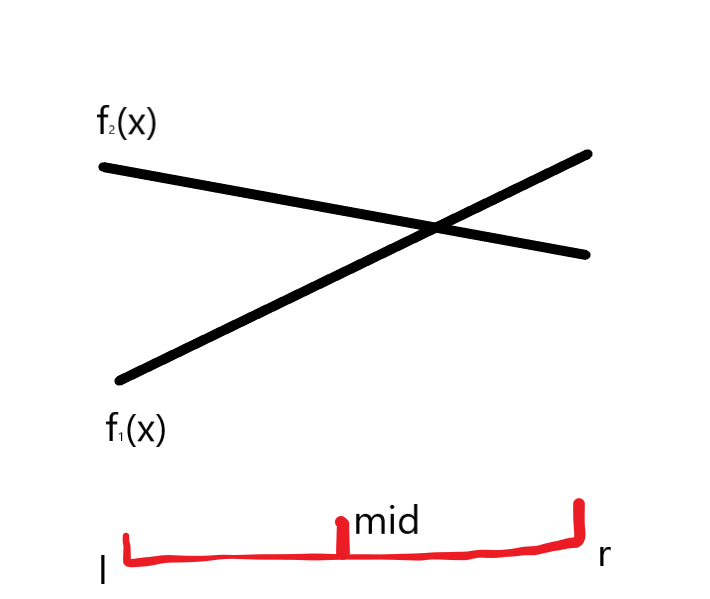# 李超树学习笔记

## 步入正题

$BUT$！这么做还有一个问题：插入线段怎么办？

1. 如果$f_1(l)\geq f_2(l),f_1(r)\geq f_2(r)$：2. 如果$f_1(l)\leq f_2(l),f_1(r)\leq f_2(r)$：3. 如果$f_1(l)\geq f_2(l),f_1(r)\leq f_2(r)\text{或者}f_1(l)\leq f_2(l),f_1(r)\geq f_2(r)$：# BZOJ4515: [Sdoi2016]游戏

## 后记

posted @ 2019-04-03 00:29  符拉迪沃斯托克  阅读(506)  评论(0编辑  收藏
Live2D